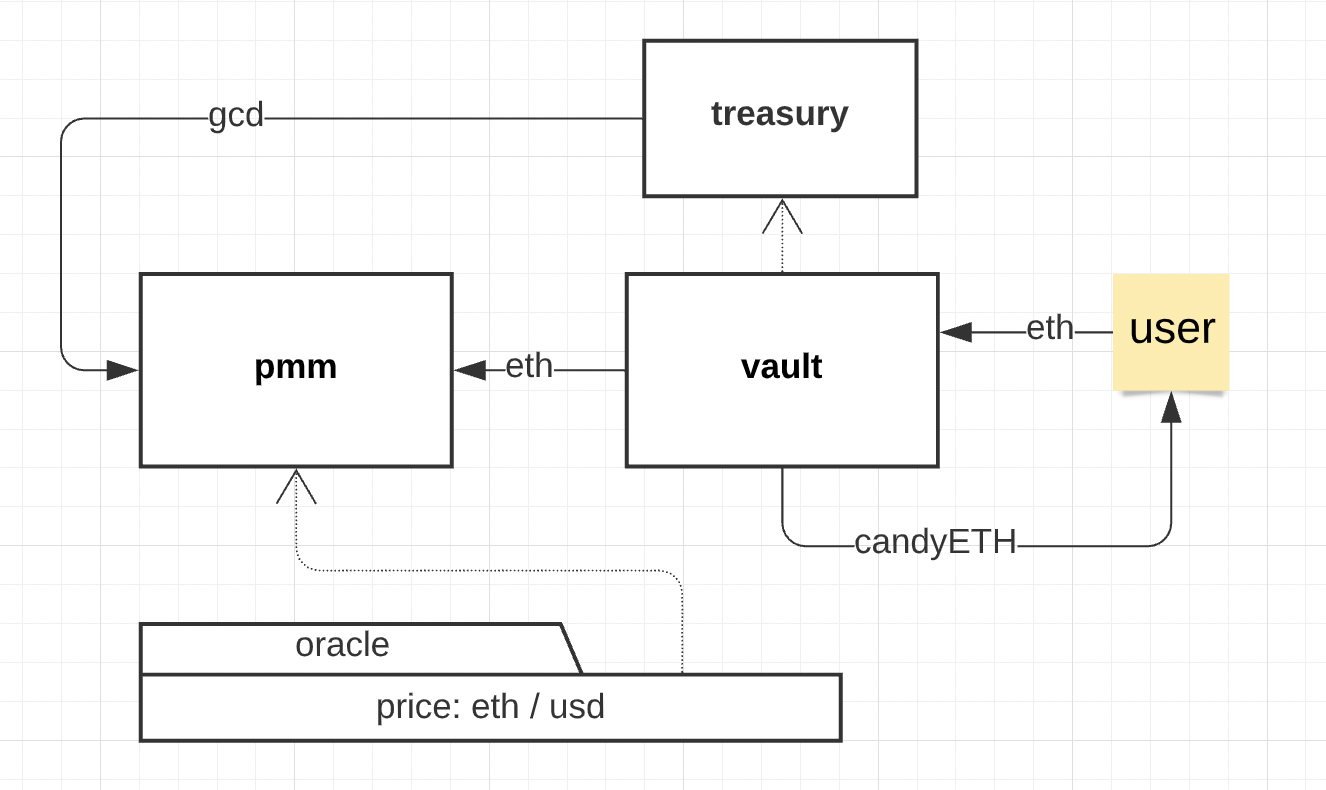🍬
CANDY
A single-sided liquidity mining and farming protocol

## Intro

With the advent of AMM DEXes, most liquidity mining tools allow for holding a pair of tokens in a pool, where impermanent loss becomes the primary risk for engaging in liquidity provision. Engaging in risky activity required from a liquidity provider is rewarded by fees shared across LPs and sometimes by extra incentives formed from the distribution of farming tokens.
A crucial law of AMMs is the more liquidity is present in a pool, the easier it is for token holders to buy and sell. In cases where protocol-owned liquidity is insufficient, assets can be rented from users in order to boost the liquidity available in pools, which can be an efficient tool to support short-term trading. By borrowing the liquidity, protocols can reduce slippage on exchanges, thereby making their tokens more marketable.
In this article, we will describe Candy, a new protocol for liquidity mining and farming which does not require putting a pair of tokens into DEX pools. Such protocols are usually called “single sided” because liquidity provision is done with only one token.
In Candy, the risk of impermanent loss is replaced by the risk of withdrawing a “blend” of originally deposited assets with a fraction of assets issued by the protocol. A similar approach is implemented in different protocols where users also provide single sided liquidity, such as Curve. The withdrawal process in such protocols yields a mixture of stablecoins in the proportion determined by the asset balance in the pool. This approach can work for stablecoins or assets with the same peg on AMMs. Candy applies similar principles to PMM pools, with the quote asset minting being controlled by the protocol or a DAO.

## Definitions

$candyETH$
- an ERC20 vault token which the user receives in exchange for locking ETH (or a token pair pegged to ETH dollar value) in Candy protocol
$GCD$
- stablecoin mintable from DAO treasury
PMM - Proactive Market Maker DEX pool
$lpETH$
- ETH locked in the PMM pool, owned by the protocol (POL)
$lpGCD$
- stablecoin locked in the PMM pool
$blend$
-
$ETH/GCD$
tokens pair; its dollar value is equal to $ETH value being put in Candy to get , and the proportion of ETH and GCD depends on the PMM liquidity balance $fraction$ - the$ share of GCD in a blend
- means the dollar value of asset X

## Algorithm

1. 1.
Alice deposits 10 ETH and receives 10
$candyETH$
. ETH price is $100, so the total$ value deposited by Alice is $1000; 2. 2. Alice’s 10 ETH are paired with $GCD$ minted from DAO treasury and put into the PMM pool, which yields lpETH and lpGCD; 3. 3. The TVL in dollars is always equal to: In this case, =$4000 and
= $3000 4. 4. To redeem her locked funds, Alice burns 10 candyETH to exchange it back for an $ETH/GCD$ blend of 10 $ETH$ dollar value. The proportion (fraction) is determined by the formula: $Fraction(GCD)=max(1-(\frac{lpETH}{candyETH}),0)$ For example, if $lpETH = candyETH$ , Alice can exchange 10 $candyETH$ back for 10 ETH without any $GCD$ . If the pool has only 8 $lpETH$ and the total issued $candyETH$ is 10, the proportion is 0.2, therefore Alice will receive a blend of 8 $ETH$ and 200 $GCD$ , so that the total dollar value remains equal to 10 $ETH$ .## Liquidity ### Case 1: Equilibrium In this case, $lpETH$ is equal to $candyETH$ . This case is explained above, where Alice receives 10 $ETH$ back for 10 $candyETH$ . ### Case 2: Base Dominance In this case, there is more $lpETH$ than $candyETH$ . The fraction is therefore equal to 0. Similar to the above case, Alice will receive 10 $ETH$ back for 10 $candyETH$ . The corner case is $candyETH=0$ , where the pool has some left $lpETH$ and the equal$ amount of
$GCD$
.

### Case 3: Quote Dominance

In this case, there is less
$lpETH$
than
$candyETH$
. Alice receives a blend where the proportion of GCD depends on the
$1-(lpETH/candyETH)$
ratio. The corner case is
$lpETH = 0$
$ETH$
\$ value in
$GCD$
stablecoin.

## Incentives

The locked tokens (e.g. ETH), which serve as rented liquidity for the DAO, are used directly in PMM pools to support trading activity and boost the utility of DAO’s own stablecoin. The activity of liquidity providers and the risks they encounter (when fraction > 0) must be compensated by rewards coming from the DAO. There are several types of rewards possible:
• staking of candyETH tokens with a fixed APR, which comes either from governance token emission or stablecoin emission. This process is called liquidity farming.
• PMM DEX trading fees distributed between candyETH stakers.

## PMM

The PMM architecture based on asymmetric bonding curves that allows for asymmetric liquidity can easily support a high
in the pool. In the equilibrium case, the PMM pool will contain ¼ of ETH and ¾ of GCD.